Question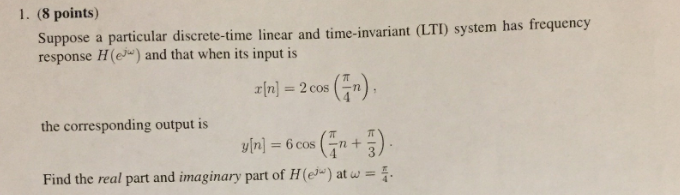Explain how fourier transform is done?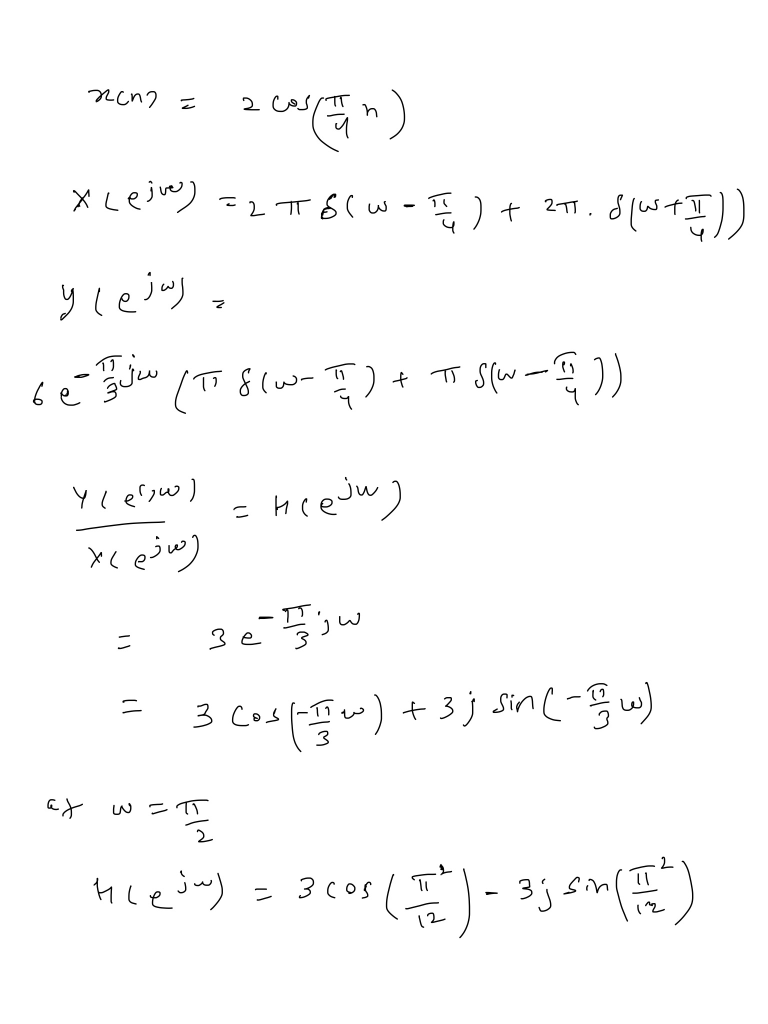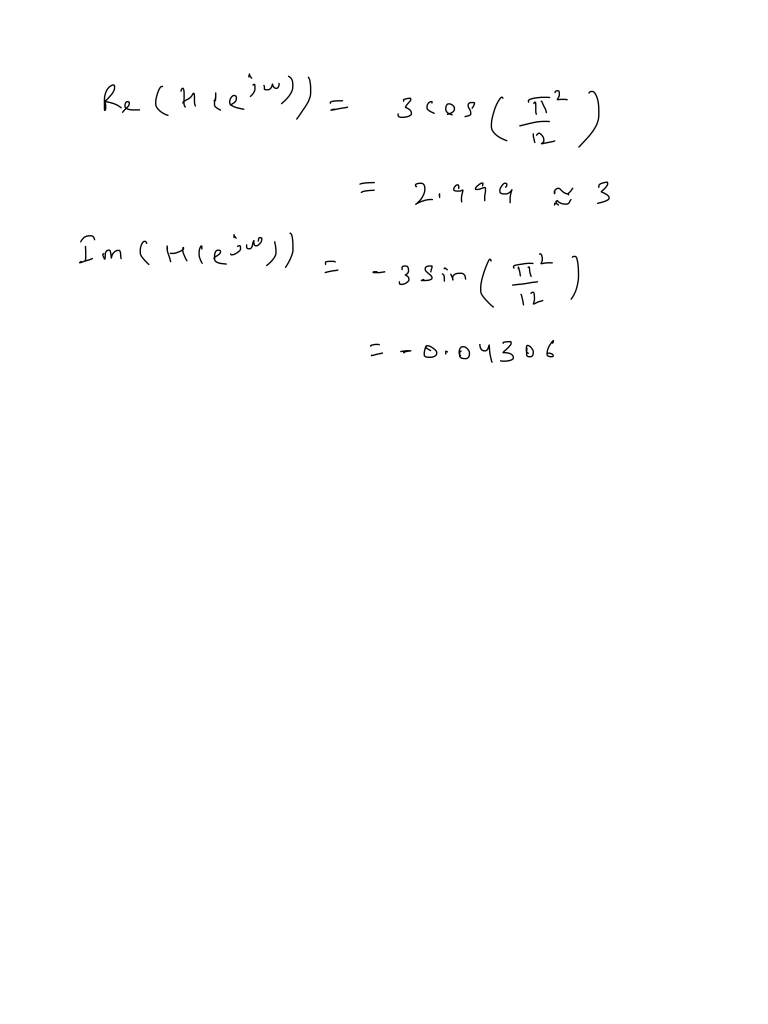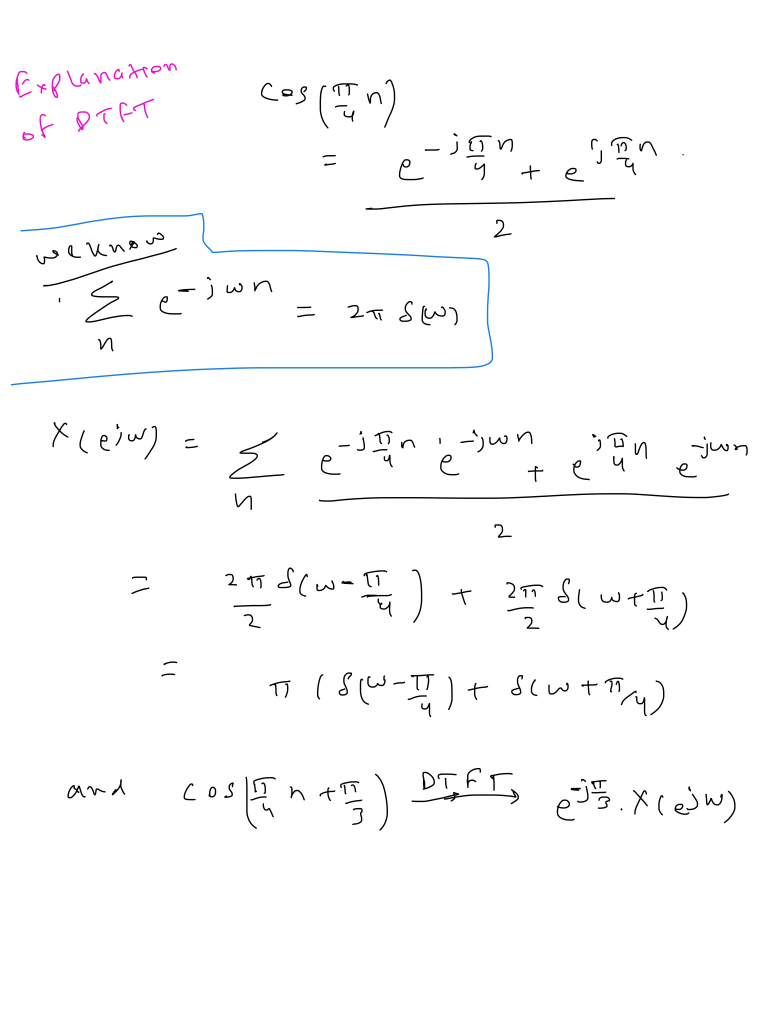#### Earn Coins

Coins can be redeemed for fabulous gifts.

Similar Homework Help Questions
• ### 4. Consider the magnitude and phase of the frequency response Hi(2) of a linear and time-invariant...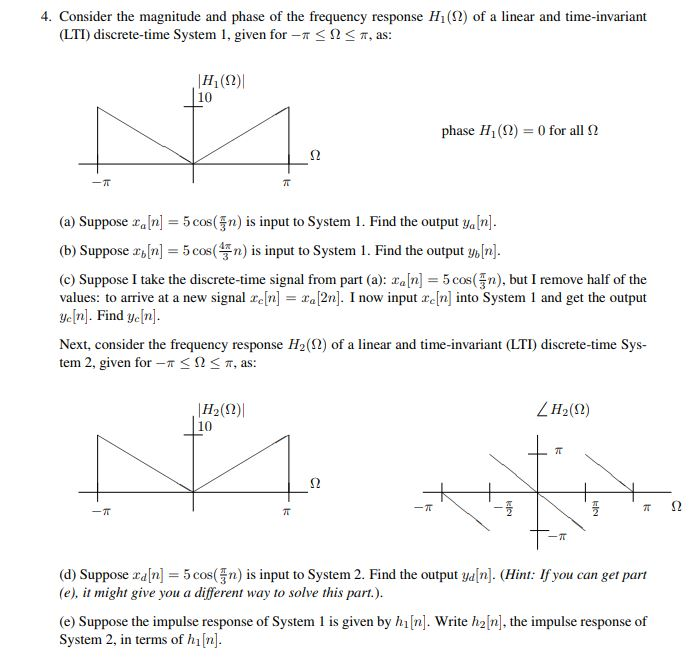4. Consider the magnitude and phase of the frequency response Hi(2) of a linear and time-invariant (LTI) discrete-time System 1, given for-r < Ω-T, as: H, (12)| 10 phase H1(Ω)--0 for all Ω (a) Suppose an 5cos(n s input to System 1. Find the output ya[n] (b) Suppose ancos(is input to System 1. Find the output ybn] (c) Suppose I take the discrete-time signal from part (a): xa[n] 5cos(n), but I remove half of the values: to arrive at a...

• ### Q8) Consider the following causal linear time-invariant (LTI) discrete-time filter with input x[n...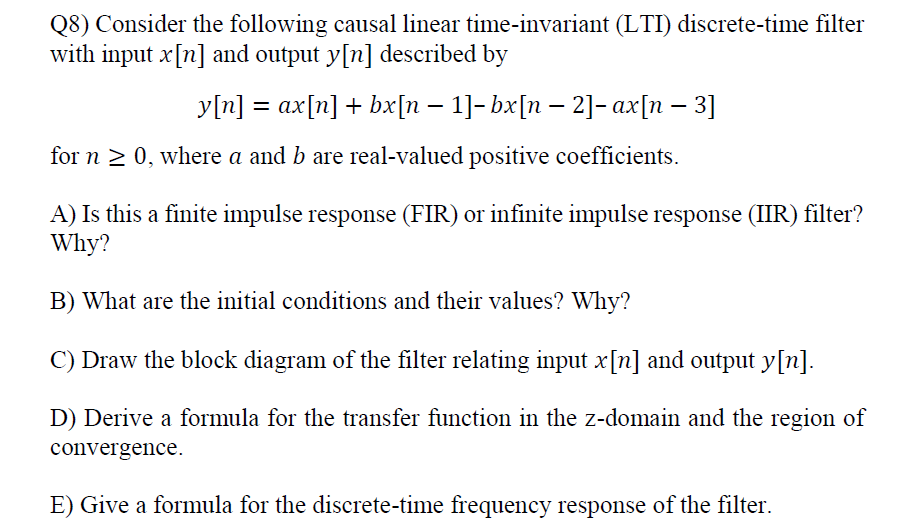Q8) Consider the following causal linear time-invariant (LTI) discrete-time filter with input x[n] and output y[n] described by bx[n-21- ax[n-3 for n 2 0, where a and b are real-valued positive coefficients. A) Is this a finite impulse response (FIR) or infinite impulse response (IIR) filter? Why? B) What are the initial conditions and their values? Why? C) Draw the block diagram of the filter relating input x[n] and output y[n] D) Derive a formula for the transfer function in...

• ### Problem 9.5 (Superposition input) A linear time-invariant system has frequency response The input...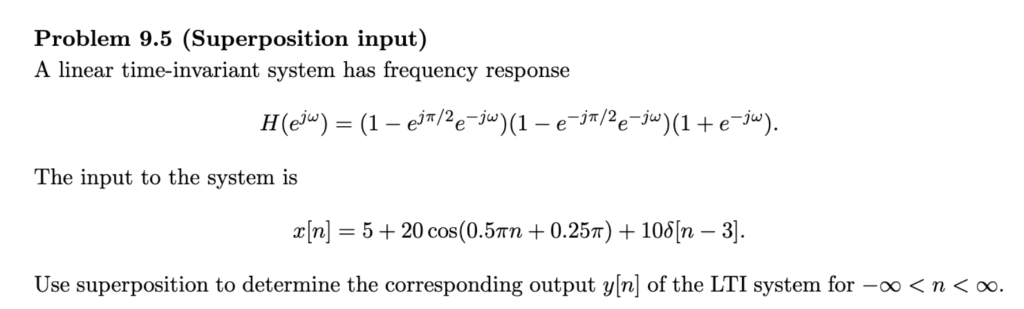Problem 9.5 (Superposition input) A linear time-invariant system has frequency response The input to the system is zin] = 5 + 20 cos(0.5mn + 0.25m) + 108[n-3]. Use superposition to determine the corresponding output vin] of the LTI system for-oo < nく00. Problem 9.5 (Superposition input) A linear time-invariant system has frequency response The input to the system is zin] = 5 + 20 cos(0.5mn + 0.25m) + 108[n-3]. Use superposition to determine the corresponding output vin] of the LTI...

• ### Consider the discrete-time periodic signal n- 2 (a) Determine the Discrete-Time Fourier Series (DTFS) coefficients ak...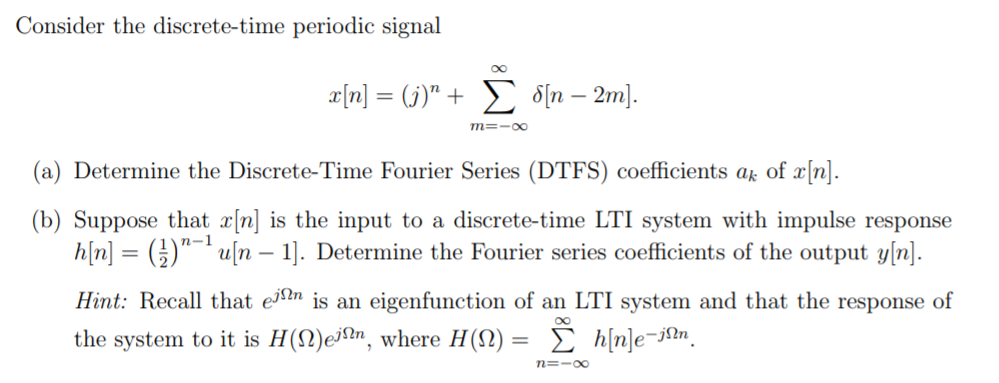Consider the discrete-time periodic signal n- 2 (a) Determine the Discrete-Time Fourier Series (DTFS) coefficients ak of X[n]. (b) Suppose that x[n] is the input to a discrete-time LTI system with impulse response hnuln - ]. Determine the Fourier series coefficients of the output yn. Hint: Recall that ejIn s an eigenfunction of an LTI system and that the response of the system to it is H(Q)ejfhn, where H(Q)-? h[n]e-jin

• ### Problem 5.3 (20 Points) A discrete-time, linear time-invariant system H is formed by ar- ranging three...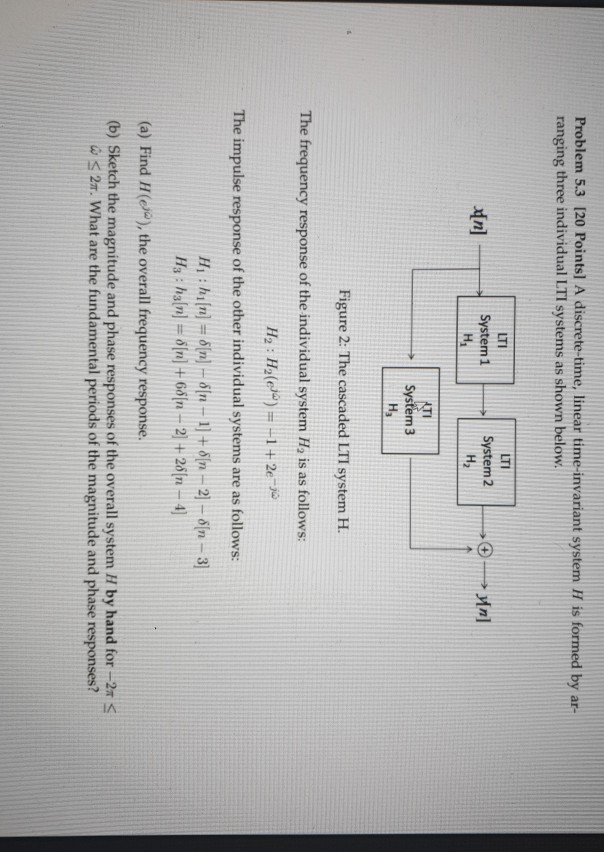Problem 5.3 (20 Points) A discrete-time, linear time-invariant system H is formed by ar- ranging three individual LTI systems as shown below. LTI LII System 1 System 2 n] > >yn] ATI System 3 Figure 2: The cascaded LTI system H. The frequency response of the individual system H, is as follows: H2 : H el) = -1 + 2e- ja The impulse response of the other individual systems are as follows: Huhn = 0[n] - [n - 1] +...

• ### 6. (15) Consider the following causal linear time-invariant (LTT) discrete-time filter with input in and output...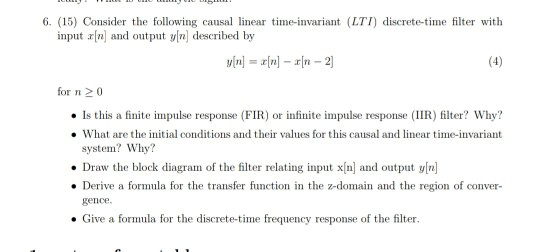6. (15) Consider the following causal linear time-invariant (LTT) discrete-time filter with input in and output yn described by y[n] = x[n] – rn - 2 for n 20 . Is this a finite impulse response (FIR) or infinite impulse response (IIR) filter? Why? • What are the initial conditions and their values for this causal and linear time-invariant system? Why? • Draw the block diagram of the filter relating input x[n) and output y[n] • Derive a formula for...

• ### Problem 1 You are given the discrete-time LTI system with impulse response, Calculate the Fourier series coefficients o...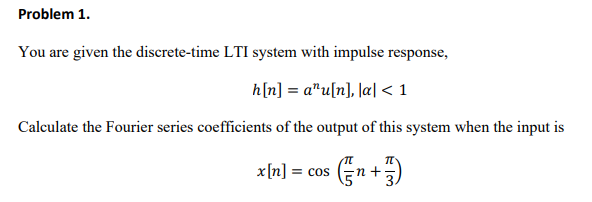Problem 1 You are given the discrete-time LTI system with impulse response, Calculate the Fourier series coefficients of the output of this system when the input is x[n] = cos(n+π) Problem 1 You are given the discrete-time LTI system with impulse response, Calculate the Fourier series coefficients of the output of this system when the input is x[n] = cos(n+π)

• ### For a continuous time linear time-invariant system, the input-output relation is the following (x(t) the input, y(t) the...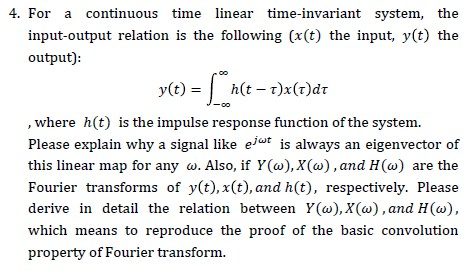For a continuous time linear time-invariant system, the input-output relation is the following (x(t) the input, y(t) the output): , where h(t) is the impulse response function of the system. Please explain why a signal like e/“* is always an eigenvector of this linear map for any w. Also, if ¥(w),X(w),and H(w) are the Fourier transforms of y(t),x(t),and h(t), respectively. Please derive in detail the relation between Y(w),X(w),and H(w), which means to reproduce the proof of the basic convolution property...

• ### Q.5 (a) Show that a linear, time-invariant, discrete-time system is stable in the bounded- input bounded-output...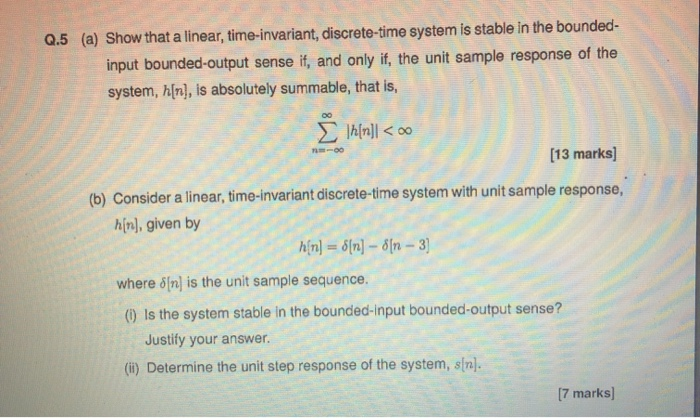Q.5 (a) Show that a linear, time-invariant, discrete-time system is stable in the bounded- input bounded-output sense if, and only if the unit sample response of the system, h[n], is absolutely summable, that is, Alfa]]<00 | [n]| < do ***** (13 marks] (b) Consider a linear, time-invariant discrete-time system with unit sample response, hin), given by hin] = a[n] – đặn – 3 where [n] is the unit sample sequence. (1) Is the system stable in the bounded-input bounded-output sense?...

• ### 4. A discrete time system is both linear and time invariant. Suppose the output due to...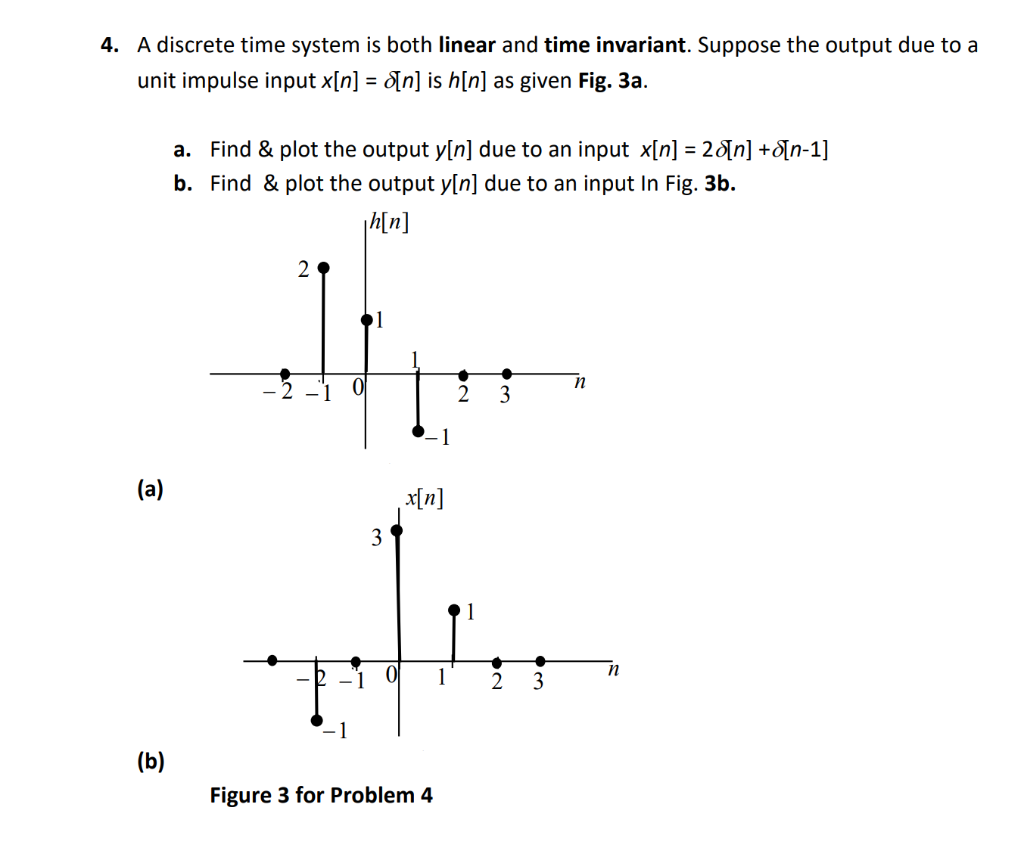4. A discrete time system is both linear and time invariant. Suppose the output due to a unit impulse input x[n] = o[n] is h[n] as given Fig. 3a. a. Find & plot the output y[n] due to an input x[n] = 28[n] + [n-1] b. Find & plot the output y[n] due to an input In Fig. 3b. hin (a) x[n] (b) Figure 3 for Problem 4